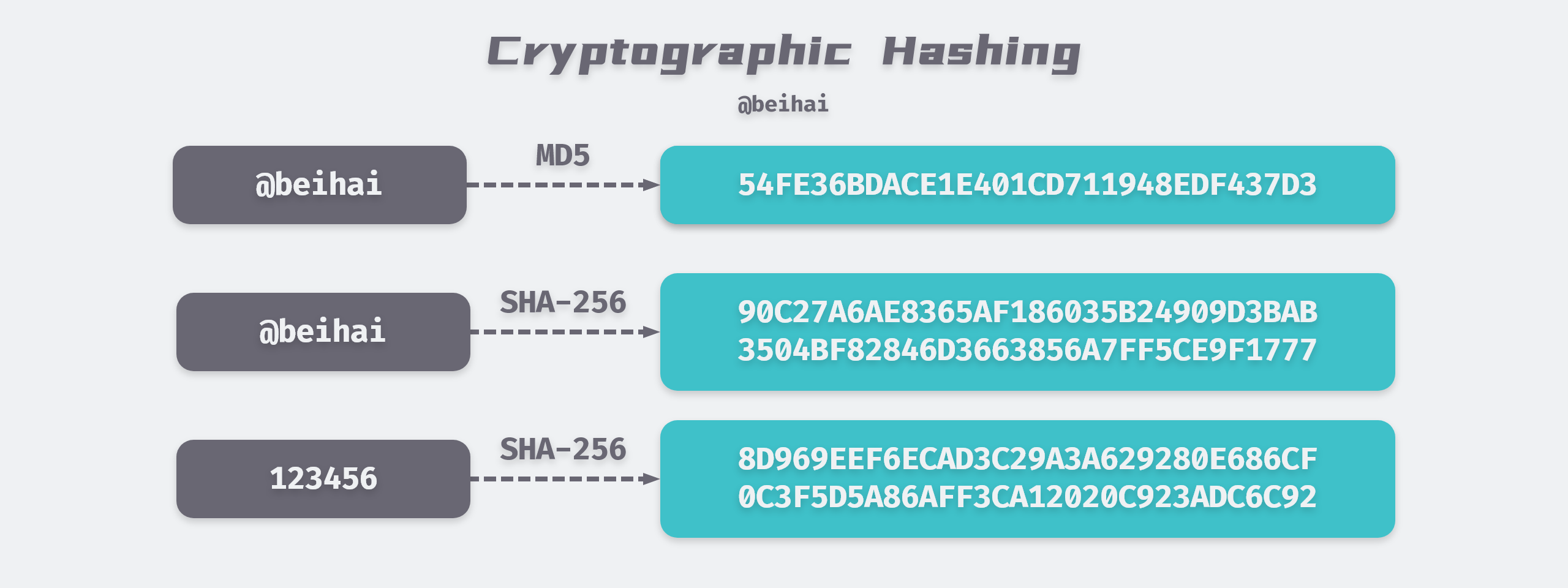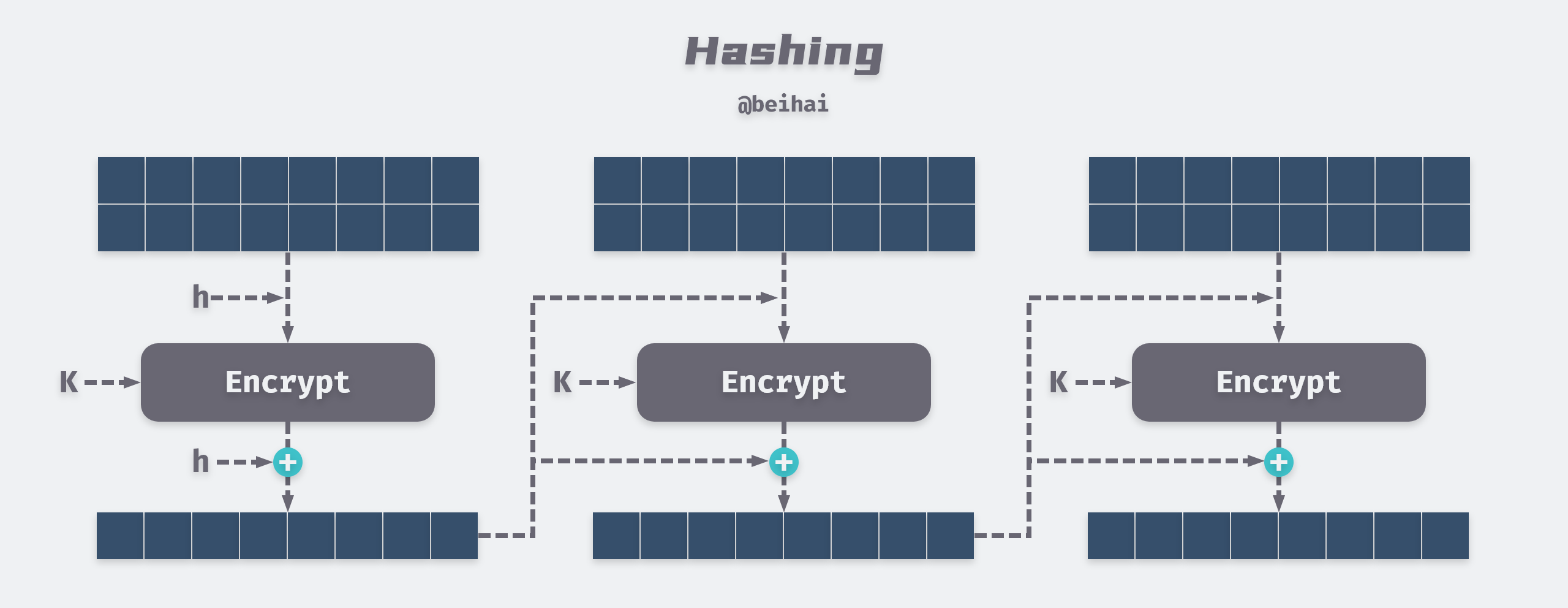Hash function, also known as a hash algorithm, is a method for creating small numerical “fingerprints” from any data (files, characters, etc.). Hash algorithms only need to satisfy the need to map a hash object to another interval, so they can be divided into cryptographic hashes and non-cryptographic hashes depending on the usage scenario.## Overview

Cryptographic hashes are considered one-way functions, meaning that it is extremely difficult to extrapolate back from the output of a hash function to what the input data is. The input data of a cryptographic hash function is usually called a message, and its output is usually called a digest. An ideal cryptographic hash function usually has the following three properties.

• Unidirectionality: it is extremely difficult to derive the original message from a known hash value.
• Uniqueness: it is infeasible to modify the content of a message without altering the hash value.
• Collision resistance: it cannot give the same hash value for two different messages.

One of the uncollidability refers to the fact that the overhead of hash collision is beyond the human acceptable level with the current level of algorithm and arithmetic power. Taking SHA-256 as an example, there are about 1077 possible hash values, while the current human estimate of the total number of atoms in the universe is about 1080. Although the probabilistic birthday paradox problem exists , the number of possible collision tests for a hash table of N-bit length is not 2N but only 2N/2 times, but it is still a huge number.

The common cryptographic hash functions are MD5, SHA-1, SHA-2 (including SHA-224, SHA-256, SHA-512, etc.). Although there are many types, the basic structure of the algorithm is the same, except for some differences in the length of the generated digest and the content of the loop body. The following is an example of SHA-256 to introduce the execution steps of cryptographic hash algorithm in detail.

## SHA-256 Implementation Principles

### Constant initialization

The SHA-256 algorithm uses 8 hash primitives and 64 hash constants, where the 8 hash primitives are the first 32 bits of the fractional part of the square root of the first 8 prime numbers (2,3,5,7,11,13,17,19) of the natural numbers.

The 64 hash constants are the first 32 bits taken for the fractional part of the cube root of the first 64 primes in the natural numbers, labeled k[t].

 ``````1 2 3 4 5 6 7 8 `````` ``````428a2f98 71374491 b5c0fbcf e9b5dba5 3956c25b 59f111f1 923f82a4 ab1c5ed5 d807aa98 12835b01 243185be 550c7dc3 72be5d74 80deb1fe 9bdc06a7 c19bf174 e49b69c1 efbe4786 0fc19dc6 240ca1cc 2de92c6f 4a7484aa 5cb0a9dc 76f988da 983e5152 a831c66d b00327c8 bf597fc7 c6e00bf3 d5a79147 06ca6351 14292967 27b70a85 2e1b2138 4d2c6dfc 53380d13 650a7354 766a0abb 81c2c92e 92722c85 a2bfe8a1 a81a664b c24b8b70 c76c51a3 d192e819 d6990624 f40e3585 106aa070 19a4c116 1e376c08 2748774c 34b0bcb5 391c0cb3 4ed8aa4a 5b9cca4f 682e6ff3 748f82ee 78a5636f 84c87814 8cc70208 90befffa a4506ceb bef9a3f7 c67178f2 ``````

These constants will be used in the algorithm loop body.

SHA-256 uses a 64-bit data to represent the length of the original message, while the message needs to be broken into 512-bit blocks during message processing, so the length of the complementary message should be an integer multiple of 512. The additional length value is divided into two steps.

1. the first bit is complemented by 1, and then all the bits are complemented by 0 until the length satisfies the remainder of 448 after modulo 512, if the length already satisfies the remainder of 448 after modulo 512, 512 bits need to be filled.
2. append a length value of 64 bits.

Take the ASCII string `abc` as an example, its hexadecimal representation is `0x616263`, and the complemented data is as follows.

 ``````1 2 `````` ``````61626380 00000000 00000000 00000000 00000000 00000000 00000000 00000000 00000000 00000000 00000000 00000000 00000000 00000000 00000000 00000018 ``````

#### main loop

Before the message digest calculation, the message needs to be decomposed into 512bit size chunks, the number of chunks is also the number of times the algorithm needs to loop. Each block will be decomposed into 16 words stored at the big end of 32bit, noted as w to w. Since 64 ‘words’ are required in the computation, the first 16 words are obtained directly from the decomposition of the i-th block of the message, and the remaining 48 words are obtained from the following iterative formula.

 ``````1 `````` ``````W[t] = Q1(W[t-2]) + W[t-7] + Q0(W[t-15]) + W[t-16] ``````

Six arithmetic functions are used in the summary calculation process.

 ``````1 2 3 4 5 6 `````` ``````Ch(x,y,z) = ((x & y) ^ ((~x) & z)) Maj(x,y,z) = ((x & y) ^ (x & z) ^ (y & z)) E0(x) = (x >> 2 | x << 30) ^ (x >> 13 | x << 19) ^ (x >> 22 | x << 10); E1(x) = (x >> 6 | x << 26) ^ (x >> 11 | x << 21) ^ (x >> 25 | x << 7) Q0(x) = (x >> 7 | x << 25) ^ (x >> 18 | x << 14) ^ (x >> 3) Q1(x) = (x >> 17 | x << 15) ^ (x >> 19 | x << 13) ^ (x >> 10) ``````

The calculation process is as follows.

 `````` 1 2 3 4 5 6 7 8 9 10 11 12 13 14 `````` ``````a~h = h0~h7 For t = 0 to 63 T1 = h + E1(e) + Ch(e,f,g) + K[t] + W[t] T2 = E0(a) + Maj(a,b,c) h = g g = f f = e e = d + T1 d = c c = b b = a a = T1 + T2 h0~h7 = a~h + h0~h7 ``````

The result of each block operation is the final a~h value plus h0~h7, and is used as the initial value of the next block, which will be output as a hash value if it is the last block.

Take the above string `abc` as an example, the algorithm flow is as follows.

 `````` 1 2 3 4 5 6 7 8 9 10 11 12 13 14 15 16 `````` `````` a b c d e f g h init: 6a09e667 bb67ae85 3c6ef372 a54ff53a 510e527f 9b05688c 1f83d9ab 5be0cd19 t = 0 5d6aebcd 6a09e667 bb67ae85 3c6ef372 fa2a4622 510e527f 9b05688c 1f83d9ab t = 1 5a6ad9ad 5d6aebcd 6a09e667 bb67ae85 78ce7989 fa2a4622 510e527f 9b05688c t = 2 c8c347a7 5a6ad9ad 5d6aebcd 6a09e667 f92939eb 78ce7989 fa2a4622 510e527f ... t = 63 506e3058 d39a2165 04d24d6c b85e2ce9 5ef50f24 fb121210 948d25b6 961f4894 H1 = 6a09e667 + 506e3058 = ba7816bf H2 = bb67ae85 + d39a2165 = 8f01cfea H3 = 3c6ef372 + 04d24d6c = 414140de H4 = a54ff53a + b85e2ce9 = 5dae2223 H5 = 510e527f + 5ef50f24 = b00361a3 H6 = 9b05688c + fb121210 = 96177a9c H7 = 1f83d9ab + 948d25b6 = b410ff61 H8 = 5be0cd19 + 961f4894 = f20015ad ``````

The above process can be represented in the following sketch. It can be seen that the digest algorithm operates by grouping blocks to continuously compress the message and finally generate the ciphertext.## Summary

As attack algorithms continue to improve and computational power increases, cryptographic hashing algorithms are gradually being ‘broken’, for example MD5 and SHA-1 have been shown to be non-collidable, i.e. `MD5(m1) == MD5(m2)`.

1. 2004, Prof. Xiaoyun Wang proved that MD5 can generate collisions: Collisions for Hash Functions.
2. 2005, Prof. Xiaoyun Wang improved the attack algorithm to find SHA-1 collisions within 263 time complexity: New Cryptanalytic Results Against SHA-1.
3. 2017, Google Inc. created two PDF files with the same SHA-1 value but different content, representing that the SHA-1 algorithm has been officially breached:Google: Announcing the first SHA1 collision.

Why is non-collidability so important to cryptographic hashing algorithms? From the implementation steps of SHA-256 algorithm, we can see that the reverse calculation of cryptographic hash is almost impossible and the cost of brute force cracking method is too high, so the so-called attack on cryptographic hash algorithm is actually using hash collision as a breakthrough for data forgery. Take the common saving user password as an example, if it is stored in plaintext, once the data leakage occurs, then all accounts will be stolen, so some of the following methods are commonly used for Hash encryption.

• Hash encryption: Hash encryption alone cannot guarantee the security of passwords because user passwords are usually short characters and can be cracked using brute force cracking or rainbow table attacks no matter which encryption algorithm is used.
• Hash with salt: Add random salt to the original message and then hash encrypt it, and save the salt with the password for the next login verification. Adding random salt increases the difficulty of rainbow table cracking, prompting attackers to give up cracking. However, if an insecure hash function (MD5) is computed on the password, after the database is compromised, the attacker can find out the collision message based on the hash value, and the message can be verified regardless of whether it is the same as the password.
• Dedicated hash function encryption: use `bcrypt` and other hash functions dedicated to password encryption for encryption, such functions usually have a long computing time, which greatly increases the cost of the attack.

Password encryption is not just a technical problem; for an attacker, even breaking an encrypted password is meaningless if the cost of breaking it is greater than the cost of gain. As for those Hash functions that are proven to generate hash collisions, the cost of attacking them is low, and the cost of cracking them keeps decreasing with the improvement of algorithms and hardware level. From the security point of view, insecure hash algorithms should be replaced in time.

Cryptographic hash is more widely used, the author studied in detail the implementation principles of hash algorithms, but also to better understand the way they are used and attacked, rather than just tuning a library of functions in programming. Before this thought that the knowledge of cryptography is relatively obscure and difficult to understand, in fact, leaving aside the intermediate mathematical operations, the overall logic is very clear, the basic structure is easy to understand.

## Reference

• `https://zh.wikipedia.org/wiki/%E6%95%A3%E5%88%97%E5%87%BD%E6%95%B8`
• `https://zh.wikipedia.org/wiki/%E5%AF%86%E7%A2%BC%E9%9B%9C%E6%B9%8A%E5%87%BD%E6%95%B8`
• `https://en.wikipedia.org/wiki/Birthday_attack`
• `https://wiki.mbalib.com/wiki/%E7%94%9F%E6%97%A5%E6%82%96%E8%AE%BA`
• `http://www.iwar.org.uk/comsec/resources/cipher/sha256-384-512.pdf`
• `https://juejin.im/post/5ce6b828f265da1bba58dd9e#heading-1`
• `https://www.jianshu.com/p/732d9d960411`
• `https://en.wikipedia.org/wiki/Bcrypt`
• `https://wingsxdu.com/posts/algorithms/cryptographic-hashing-function/`